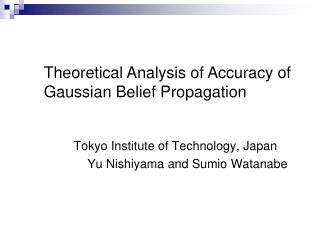# Tokyo Institute of Technology, Japan Yu Nishiyama and Sumio Watanabe - PowerPoint PPT PresentationDownload PresentationTokyo Institute of Technology, Japan Yu Nishiyama and Sumio Watanabe

Tokyo Institute of Technology, Japan Yu Nishiyama and Sumio WatanabeDownload Presentation## Tokyo Institute of Technology, Japan Yu Nishiyama and Sumio Watanabe

- - - - - - - - - - - - - - - - - - - - - - - - - - - E N D - - - - - - - - - - - - - - - - - - - - - - - - - - -
##### Presentation Transcript

1. Tokyo Institute of Technology, Japan Yu Nishiyama and Sumio Watanabe Theoretical Analysis of Accuracy of Gaussian Belief Propagation

2. Background Belief propagation (BP) The algorithm which computes marginal distributions efficiently Marginal distribution: requires huge computational cost.

3. Variety of Research Areas ex. (i) Probabilistic inference for AI (ii) Error correcting code (LDPC, Turbo codes) noise correcting 000101 000111 000101 (iii) Code division multiple access (CDMA) (iv) Probabilistic image processing restore degrade image restored image

4. Properties of BP & Loopy BP (LBP) Tree-structured target distribution Exact marginal probabilities Loop-structured target distribution Convergence? Approximate marginal probabilities Ex. T. Heskes, ”On the Uniqueness of Loopy Belief Propagation Fixed Points”, Neural Computation16(11), 2379-2414, 2004. Y. Weiss,”Correctness of belief propagation in graphical models with arbitrary topology”, Neural Computation13(10), 2173-2200, 2001.

5. Purpose We analytically clarify the accuracy of LBP when the target distribution is a Gaussian distribution. What is the conditions for LBP convergence? How close is the LBP solution to the true marginal distributions? In Probabilistic image processing K. Tanaka, H. Shouno, M. Okada, “Accuracy of the Bethe approximation for hyperparameter estimation in probabilistic image processing”, J.phys. A, Math. Gen., vol.37, no.36, pp.8675-8696, 2004.

6. Table of Contents ・BP ＆ LBP ・Gaussian Distribution ・Main Results (i) Single Loop (ii) Graphs with Multi-loops ・Conclusion

7. Graphical Models Target distribution Marginal distribution

8. BP ＆ LBP Marginal distribution

9. If it converges How are messages decided? are decided by the fixed-points Messages of a message update rule: a fixed-point

10. Gaussian Distribution Target distribution: ( Inverse covariance matrix) Messages: Update rule:

11. Fixed-Points of Messages Theorem1 When a Gaussian distribution forms a single loop, the fixed-points of messages are given by Single loop are the cofactors. where

12. LBP Solution Theorem 2 The solution of LBP is given by where

13. True Loopy Belief Propagation Belief Propagation Intuitive Understanding LBP Solution Loop Tree

14. Accuracy of LBP Theorem 3 The Kullback-Leibler (KL) distances are calculated as True marginal density Solution of LBP where is given by Convergence condition is since

15. Graphs with Multi-Loops How about the graphs having arbitrary structures? We clarify the LBP solution at small covariances. Multi-loops We derive the expansions w. r. t. where inverse covariance matrix is

16. A Fixed-Point of Inverse Variances Theorem 4 A fixed-point of inverse variances satisfies the following system of equations: The solution of the system is expanded as

17. Comparison with true inverse variances Expansions of LBP solution are True inverse variances are

18. Accuracy of LBP Theorem 5 The Kullback-Leibler (KL) distances are expanded as True marginal density Solution of LBP where are

19. Conclusion (i) For a single loop, we revealed the parameter that determines the accuracy of LBP and the condition that tells us when LBP converges. (ii) For arbitrary structures, we revealed the expansions of LBP solution at small covariances and the accuracy. These fundamental results contribute to understanding the theoretical properties underlying LBP. We analytically clarified the accuracy of LBP in a Gaussian distribution.